HOME COURSES PREVIEW REVIEW ABOUT CONTACTTOLL-FREE INFO & ORDERING: M-F: 9am-5pm (PST): (877) RAPID-10

 Quick Search: Keywords:

 Rapid Learning Member Area:Note: If you are a legacy user of chemistry24 members, please request a new login access to the premium server with your full name and old login email via vip@rapidlearningcenter.com

 Rapid Courses Catalog : Physics in 24 Hours Chemistry in 24 Hours Biology in 24 Hours Mathematics in 24 Hours

Weekly Physics Tips:
Want to become a top gun in your class? How about study less yet score high? Sign up this Physics Survival Weekly to learn how. Designed specifically for students who are taking physics, this free newsletter will show you how to survive and excel in class! Weekly topics include:
• How to Study Physics Effectively
• How to Read Physics Textbooks Easily
• How to Solve Physics Problems Systematically
• How to Score High on Physics Exams Strategically
• How to Master Physics Rapidly
Each week, you will receive study tips on the topics above and visual tutorial or study template to enhance your physics learning. Enter your name and email below to subscribe free:

 Physics Study Lounge These study sheets are for quick review on the subjects. Refer to our rapid courses for comprehensive review.     - Basic Skills in Physics     - Basic Math for Physics     - How to Solve Physics Problems     - Newton's Laws Study Guide     - ElectroMagnetism Quick Review     - Atomic Physics At-A-Glance     - Core Concepts in Nuclear Physics     - Special Relativity Overview

 Other Related Sites
 Note: For course links to launch, disable popup blockers or hold the ctrl key while clicking the link.Home » College Calculus

Geometry Review

 Topic Review on "Title": Angles: An angle is either the figure formed by two rays starting from a common point or the measure of such a figure. Complimentary angles are two angles whose sum is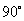. Supplementary angles are two angles whose sum is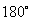. Congruent angles are angles with the same angle measurement. Vertical angles are angles at an intersection of two lines that are not adjacent to each other. Interior angles are two angles that are in the interior of a polygon. Exterior angles are two angles formed by the side of a polygon and an adjacent extends side or two angles on the outside of two parallel lines. Pythagorean Theorem: The Pythagorean Theorem is the sum of the squares for the legs of a right triangle equals the square of the hypotenuse, or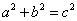. 30-60-90 Right Triangles: The sides of 30-60-90 right triangles are in the ratios of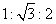. 45-45-90 Right Triangles: The sides of 45-45-90 right triangles are in the ratios of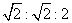. Regular polygon: A regular polygon is a geometric figure with three or more sides in which all interior angles are congruent. Area: Area is the size of a planar region or surface of a solid. Volume: Volume is the amount of space taken up by or enclosed by an object.

Rapid Study Kit for "Title":
 Flash Movie Flash Game Flash Card Core Concept Tutorial Problem Solving Drill Review Cheat Sheet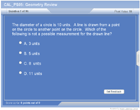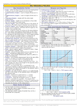"Title" Tutorial Summary : This tutorial deals with the most important geometrical concepts and their applications. Figures and relations used in geometry are covered so a deeper understanding of the ideals could be accomplished. Area and volume principles are discussed as they are applied to triangles and circles. Similarity principles are introduced with the help of 45-45-90 and 30-60-90 right triangles. Area and volume formulas are exhibited with the help of examples.

 Tutorial Features: Specific Tutorial Features: Step by step mathematical examples showing all the steps covering the Pythagorean theorem, 30-60-90 right triangles, 45-45-90 triangles, sum of interior angles, and area of complex shapes. Series Features: Concept map showing inter-connections of new concepts in this tutorial and those previously introduced. Definition slides introduce terms as they are needed. Visual representation of concepts Animated examples—worked out step by step A concise summary is given at the conclusion of the tutorial.

 "Title" Topic List: `Angles Angles and their definition Relationship of angles and their use Vertical angles Interior and exterior anglesGeometric Shapes and Figures Right triangles Pythagorean Theorem 30-60-90 right triangles 45-45-60 right triangles 45-45-90 right trianglesRegular polygonsArea and Volume Definition of area and volume Area Formulas Volume Formulas`

See all 24 lessons in college calculus, including concept tutorials, problem drills and cheat sheets:
Teach Yourself College Calculus Visually in 24 Hours